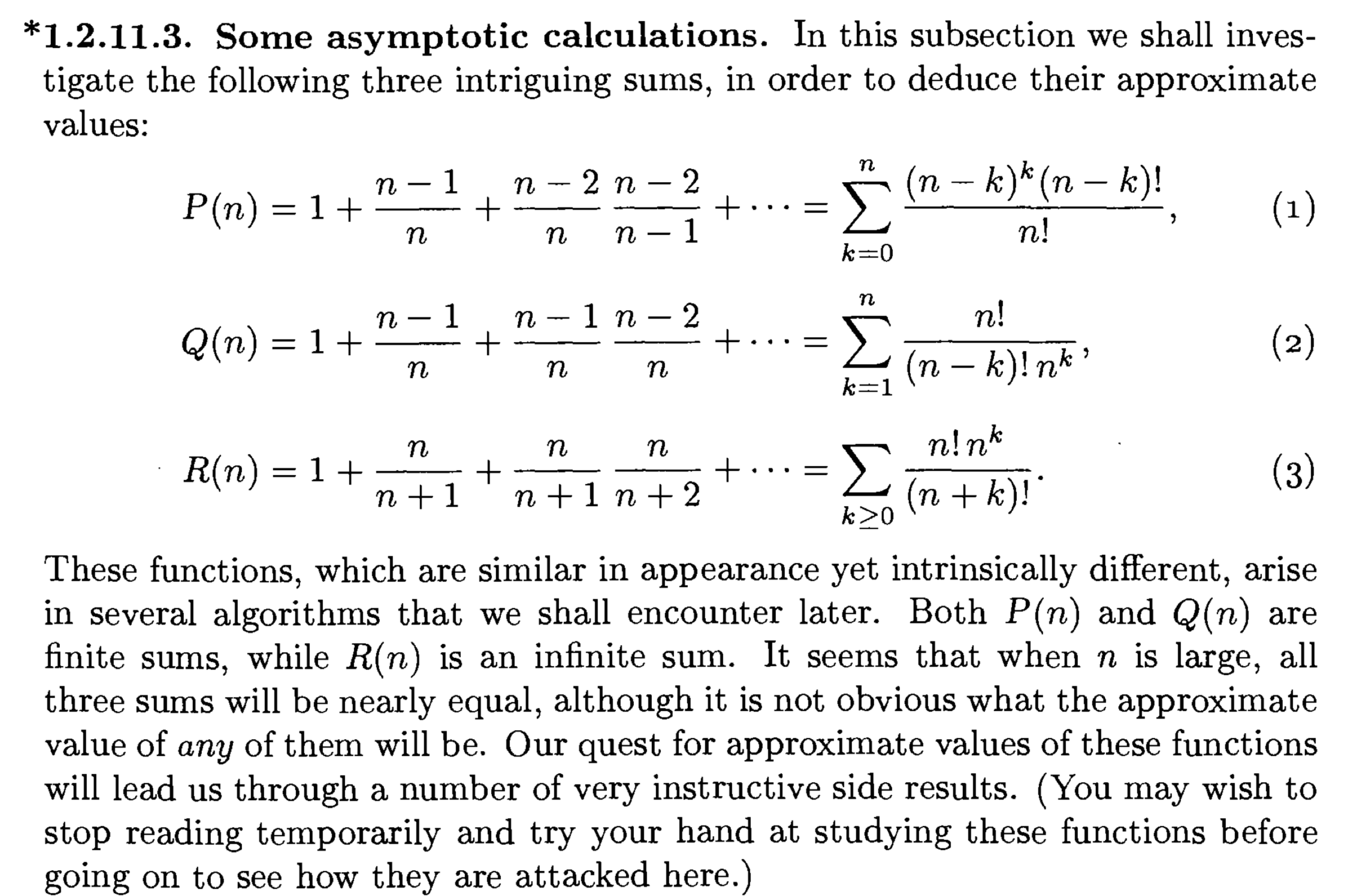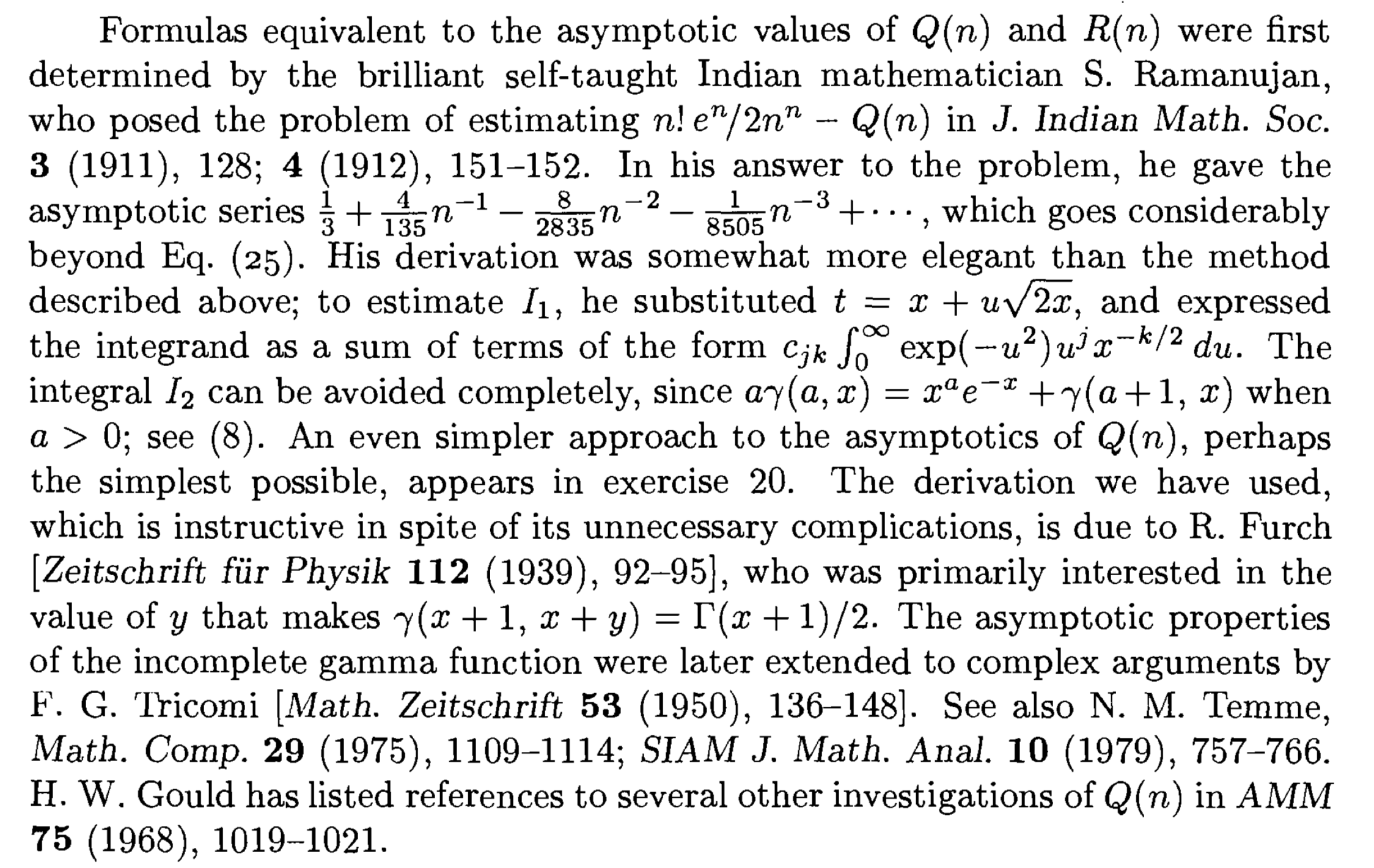# An expansion from Ramanujan related to birthday problem

A friend designed a drinking game with a lucky wheel of 30 distinct icons. When playing, each one takes turn to spin the wheel, and write down the items until the first one who gets the item that has already appeared then he drinks. And he asked what the average (expectation) of turns is. The formula is not so difficult to get but it is quite unpractical to compute the value. So I was thinking about an asymptotic formula.

Luckily, I found this here: See section 5.6, "average number of people".

My question is how to prove the Ramanujan's formula given there,

$$Q(M)=\sum_{k=1}^{M} \frac{M!}{(M-k)! M^k}\sim\sqrt{\frac{\pi M}{2}}-\frac{1}{3}+\frac{1}{12}\sqrt{\frac{\pi}{2M}}-\frac{4}{135M}+\cdots$$

Any good reference available?

We have $$Q(n)=\sum_{k=0}^{n-1} (1-1/n)(1-2/n)\ldots(1-k/n).$$ Write $$1-x/n=e^{-x/n-x^2/(2n^2)-\ldots}$$, then $$Q(n)=\sum_{k=0}^{n-1} \exp\left(-\frac{k(k+1)}{2n}-\frac{k(k+1)(2k+1)}{12n^2}-\ldots\right).$$ If $$k\geqslant n^{1/2+0.00001}$$, the corresponding summands are super-polynomially small and do not rely on the asymptotics. For $$k , the first few summands in the expansion define it with good accuracy ($$n$$ to any prescribed negative power) and we get a standard problem of the asymptotics of $$\sum_k g(k)$$ for $$g(k)=\exp(-\frac{k(k+1)}{2n}-\frac{k(k+1)(2k+1)}{12n^2})$$, say. It is obtained by Euler–Maclaurin. The main term comes from the approximation of $$\sum_k \exp(-k^2/(2n))$$ by $$\int_0^\infty \exp(-x^2/(2n))dx=\sqrt{\pi n/2}$$.